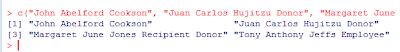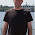## Friday, July 29, 2011

### Splitting Vectors of Uneven Strings

Suppose you have a vector of names such that the first three words in the vector contain relevant information, but there is a bunch of extraneous stuff. For example,Our goal is to collapse the first three words into one contiguous string (without the spaces) and we want to discard the extra words (because they're extraneous infromation). For a situation like this, my first thought is to use the strsplit() command, which splits vectors of strings by any specified character pattern you wish. For example, suppose that an object named myvec contains the vector of strings we would like to split into separate words. Then, the command

strsplit(myvec, " ")

produces a list of vectors of words. This is a little cumbersome because the vectors have a different length for each element in the list. Fortunately, all we want in this application is the first three words concatenated together, so some trimming will still give us the right answer. Even better, if you don't mind seeing an error message on account of trying to bind vectors of uneven lengths, we can rbind the list together using do.call() as follows:

do.call(rbind, strsplit(myvec, " "))

This still works because R recycles. For our example, we then obtain a fabulous 4x5 matrix of names:Store this matrix in the object namemat, then reduce this matrix to containing only the first three words by selecting those columns:

namemat = namemat[, 1:3]

Think of each row of this resulting matrix as a vector. If we can paste this vector together into one string, we're done. Do this using the paste() command with collapse = "". To paste() simultaneously for each row, I like to use apply. The resulting command that goes from namemat to vector of cleaned-and-concatenated strings is:

apply(namemat, MARGIN = 1, FUN = function(x){ paste(x, collapse="")})

MARGIN = 1 is for rows. Putting the pieces together, the code we developed is:

namemat = do.call(rbind, strsplit(myvec, " "))
namemat = namemat[, 1:3]
apply(namemat, MARGIN = 1, FUN = function(x){ paste(x, collapse="")})

And, this should work if your list of names is 4 lines long or 40 million lines long. That said, there was a lot of heavy lifting along the way (we created a list, collapsed a list, produced an error message as a byproduct of binding the list into a matrix, pasted each row using the collapse option and pasted quickly using apply).

There's a strange way to do this same task that doesn't require the same heavy lifting (just different heavy lifting). Use write.table() and read.table(). Start by writing the unclean initial vector to a text file without row names.

write.table(myvec, "C://vec.txt", sep = "", quote = FALSE, row.names = FALSE)

Also, turn off quoting. We do this to trick R into thinking that the spaces we want to eliminate are space delimiters. Then, read in the file using read.table() and a space delimiter.

namemat = read.table("Data/Raw/crspnames.txt", skip=1, sep = " ", quote = "", fill = TRUE)

skip =1 is to avoid reading in the variable name. The fill = TRUE argument fills in the matrix so that read.table() doesn't return an error for trying to read a non-square table. Each row of the resulting matrix namemat has a full name to be collapsed, one word per column (similar to namemat from the previous method). To complete the task, we still need to paste the first three columns together for each row. For this, use the apply-the-paste technique we used in the first method.

On a final note, if we don't mind pasting the entire vector together without the spaces (including the extraneous stuff), the easy solution is to use the gsub() command to replace spaces with nothing.

gsub(" ", "", myvec)

This method will produce an answer that is close to the one that we wanted and with a simple one line of code. Depending on your objective, this may be the best way to go -- that is, if you don't care about having extra words appended to each string.

1.How's this for doing it in one line?

do.call(rbind,lapply(myvec, FUN=function(x) paste(strsplit(x," ")[][1:3], collapse="")))

2.Clever solution!

3.you better use regexp:
eg:
myvec <- sub("([[:alpha:]]+[[:blank:]][[:alpha:]]+[[:blank:]][[:alpha:]]+)(.+)","\\1",myvec)
myvec <- gsub(" ","",myvec)

4.This is actually even shorter:
sub("(([[:alpha:]]+[[:blank:]]){3})(.+)","\\1",myvec)

5.When myvec is really big, so that each string could be millions of characters long, you don't want to work on all the things that aren't the first 3 words. Because of this, strsplit, and regular expression that involve (.+) will be slow.

A fast solution in that case is:
substr( myvec,1,attr(regexpr("[^ ]* [^ ]* [^ ]* ", myvec),"match.length"))

Here:
> myvec=rep( paste( rep(c(1:10," "),10^5),collapse=""),10)
> system.time({ substr( myvec,1,attr(regexpr("[^ ]* [^ ]* [^ ]* ", myvec),"match.length"))})
user system elapsed
0.026 0.000 0.027
> system.time( { sub("(([[:alpha:]]+[[:blank:]]){3})(.+)","\\1",myvec)})
user system elapsed
3.146 0.019 3.181
> system.time( { do.call(rbind,lapply(myvec, FUN=function(x) paste(strsplit(x," ")[][1:3], collapse="")))})
user system elapsed
2.522 0.010 2.558

6.That's a nice *string* of comments.